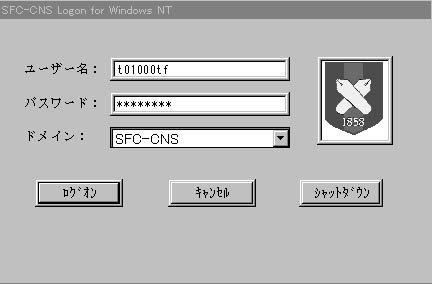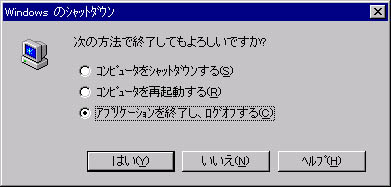Windows\$B\$NA`:n(B/Windows\$B\$N4pK\A`:n(B/\$B%m%0%*%s\$H%m%0%*%U(B(WindowsNT)

WindowsNT\$B\$G\$O(BUNIX\$B4D6-\$N%3%s%T%e!<%?\$HF1\$8\$h\$&\$K%f!<%6G'>Z\$r9T\$&I,MW\$,\$"\$j\$^\$9!%\$^\$?!\$(BWindowsNT\$B\$G\$OMxMQ3+;O\$NZ\$,9T\$o\$l!\$<+J,\$N4D6-\$rMxMQ\$G\$-\$^\$9!%(B

## 2.2.1\$B%m%0%*%s(B

\$B

1.
`\$B%m%0%*%s\$9\$k\$K\$O!\$(BCtrl + Alt + Del\$B%-!<\$r2!\$7\$F\$/\$@\$5\$\$(B'\$B\$H=q\$+\$l\$?%&%#%s%I%&\$r3NG'\$7!\$(B<CTRL>\$B!\$(B<ALT>\$B!\$(B<DEL>\$B\$rF1;~\$K2!\$9!%(B
2.
\$B?^(B2.3\$B\$h\$&\$J%&%#%s%I%&\$,I=<(\$5\$l\$k\$N\$G!\$(B`\$B%f!<%6L>(B'\$BMs(B \$B\$K(BCNS \$B\$N%m%0%\$%sL>\$r!\$(B`\$B%Q%9%o!<%I(B'\$BMs\$K(BCNS \$B\$N%Q%9%o!<%I\$rF~NO\$9\$k!%(B
3.
`[`\$B%m%0%*%s(B`]`\$B\$r%/%j%C%/\$9\$k!%(B## 2.2.2\$B%m%0%*%U(B

\$B

1.
\$B%9%?!<%H%a%K%e!<\$+\$i(B[\$B%7%c%C%H%@%&%s(B]\$B\$rA*Br\$9\$k!%(B
2.
\$B?^(B2.3\$B\$N\$h\$&\$J%&%#%s%I%&\$,I=<(\$5\$l\$k\$N\$G(B`\$B%"%W%j%1!<(B \$B%7%g%s\$r=*N;\$7!\$%m%0%*%U\$9\$k(B'\$B\$K%A%'%C%/\$r\$9\$k!%(B
3.
`[`\$B\$O\$\$(B`]`\$B\$r%/%j%C%/\$9\$k!%(B